AP State Syllabus AP Board 6th Class Maths Solutions Chapter 8 Basic Geometric Concepts InText Questions and Answers.

## AP State Syllabus 6th Class Maths Solutions 8th Lesson Basic Geometric Concepts InText Questions(Page No. 111)

i) How many rays are there ?
Solution:
Four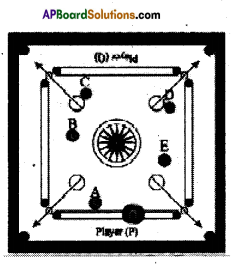ii) How many coins are close to player Q ?
Solution:
B, C and D coins are close to player Q.

iii) While striking with striker there is a possibility of a coin touches with any other. Draw all such possibilities in the given picture by means of the line segments.
Solution:
$$\overline{\mathrm{CB}}$$ and $$\overline{\mathrm{DE}}$$ .

iv) How many such line segments can be drawn in the picture ?
Solution:
$$\overline{\mathrm{AB}}, \overline{\mathrm{BC}}, \overline{\mathrm{ED}}, \overline{\mathrm{AC}}, \overline{\mathrm{AE}}, \overline{\mathrm{CD}}, \overline{\mathrm{CE}}, \overline{\mathrm{AD}}, \overline{\mathrm{BD}} \text { and } \overline{\mathrm{BE}}$$Let’s Do (Page No. 112)

Question 1.
Observe the table and their notations and fill the gaps.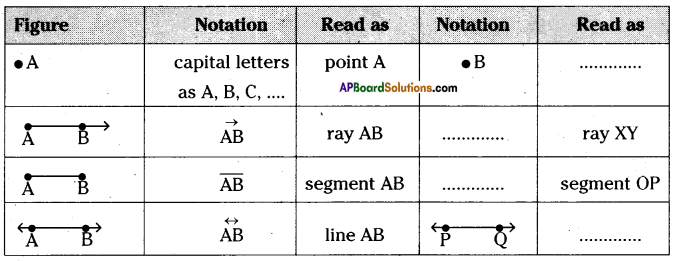Solution: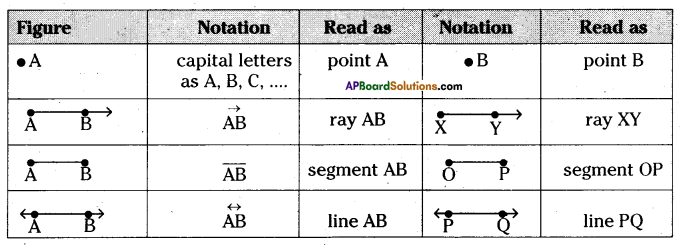Lets Explore (Page No. 114)

Question 1.
Measure the lengths of all line segments in the given figures by using divider and scale. Then compare the sides of the given figures.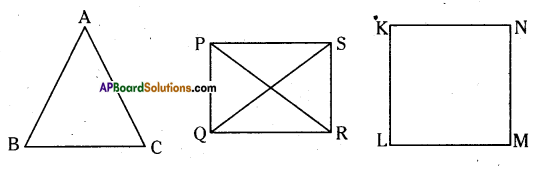Solution:
i) In ΔABC; $$\overline{\mathrm{AB}}$$ = 2.2 cm; $$\overline{\mathrm{BC}}$$ = 2 cm and $$\overline{\mathrm{AC}}$$ = 2.2 cm ‘
2.2 cm = 2.2 cm > 2 cm i.e., $$\overline{\mathrm{AB}}=\overline{\mathrm{AC}}>\overline{\mathrm{BC}}$$
Two sides are equal and one side is different in length.

ii) In PQRS rectangle $$\overline{\mathrm{PS}}=\overline{\mathrm{QR}}$$ = 2.7 cm; $$\overline{\mathrm{P Q}}=\overline{\mathrm{RS}}$$ = 1.8 cm and $$\overline{\mathrm{PR}}=\overline{\mathrm{QS}}$$ = 3.2 cm.
Opposite sides are equal and diagonals are equal in length,

iii) In KLMN square $$\overline{\mathrm{KL}}=\overline{\mathrm{LM}}=\overline{\mathrm{MN}}=\overline{\mathrm{KN}}$$ = 1.8 cm
All sides are equal in length.Let’s Do (Page No. 115)

Question 1.
(i) Find the parallel lines in the below figure. Name, write and read them.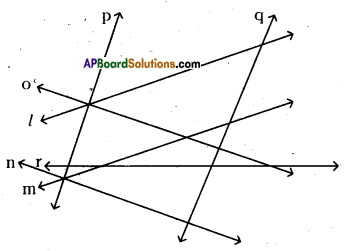Solution:
a) l, m are parallel lines.
We denote this by writing l || m and can be read as l is parallel to m.
b) n, o are parallel lines.
We denote this by writing n||o and can be read as n is parallel to o.
c) p, q are parallel lines.
We denote this by writing p||q and can be read as p is parallel to q.

ii) Find the intersecting lines in the above figure. Name, write and read them.
Solution:
Intersecting lines are (l, q); (m,q); (m, r); (n,q); (p, r); (o, r); (o, q); (q, r);

iii) Find the concurrent lines in the above figure. Name, write and read them.
Solution:
Three or more lines passing through the same point are called concurrent lines. Concurrent lines are (l, o, p) & (m, n, p).

iv) Find the perpendicular lines in the above figure. Name, write and read them.
Solution:
a) p, o are perpendicular lines.
We denote this by writing p ⊥ o and can be read as p is perpendicular to o.
b) p, n are perpendicular lines.
We denote this by writing p ⊥ n and can be read as p is perpendicular to n.
c) n, q are perpendicular lines.
We denote this by writing n ⊥ q and can be read as n is perpendicular to q.
d) q, o are perpendicular lines.
We denote this by writing q⊥ o and can be read as q is perpendicular to o.(Page No. 119)

Question 1.
Measure the angles at the vertices.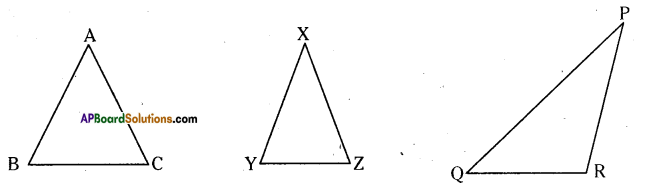Solution: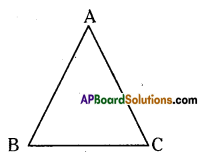In triangle ABC,
m∠BAC = 60°
m∠ABC = 60°
m∠ACB = 60°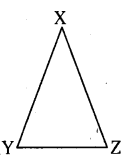In triangle XYZ,
m∠YXZ = 40°
m∠XYZ = 70°
m∠XZY = 70°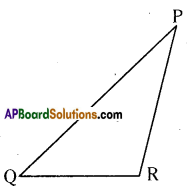In triangle PQR,
m∠QPR = 35°
m∠PQR = 38°
m∠PRQ = 107°